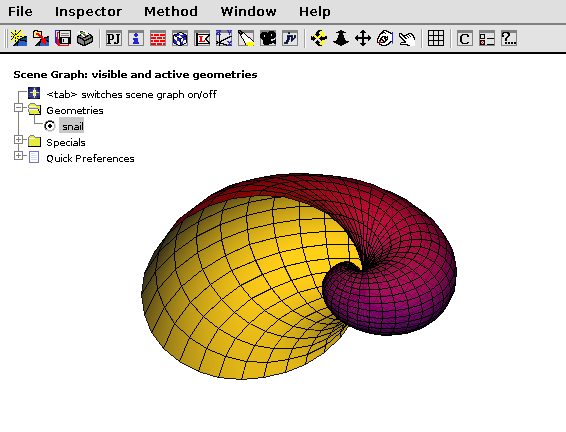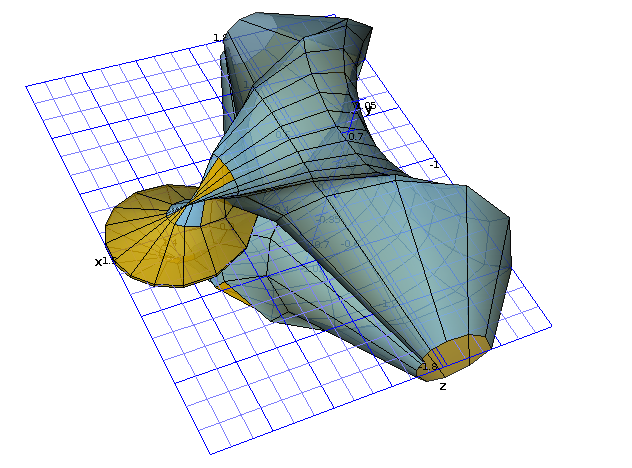DMelt:Plots/JavaView

(diff) ← Older revision | Latest revision (diff) | Newer revision → (diff)
Limitted access. First login to DataMelt if you are a full DataMelt member. Then login to HandWiki as a user.

Using JavaView

3D geometry viewer and a mathematical visualization software, called JavaView (http://www.javaview.de/) can be used with the classjhplot.HJavaView. It should be noticed that the license of JavaView should be put to the file "lib/javaview/rsrc/jv-lic.lic" relative to DataMelt installation. Without this license, the program is fully functional but you will see a message dialog about the license.

The package allows:

Visualize and study your own geometry models. The data files may reside on your local computer or somewhere on the internet. Simply browse your local disk or type the URL of a model using a web form.

• Solve ordinary differential equations interactively.
• Calculate the zero points of functions.
• Measure distances on curved polyhedral surfaces using straightest or shortest geodesic lines.
• Computes algebraic surfaces given as zero set of a polynomial function.

DataMelt allows programming and visualizing 3D mathematical objects using the Python language (as well as Groovy, Ruby). Here is a Jython example that shows a snail in 3D interactive viewer:The code is shown below:

One can draw several 3D objects which belong tojv.geom.PgElementSet class.

Showing functions in 3D

Here is an example which illustrates how to calculate parametric functions. Here is an example that illustrates how to create Kuen SurfaceThe code is shown below uses thejvx.surface.PgParmSurface class:

DataMelt supports drawing all JavaView classes that inherent from the Java classjv.project.PgGeometry. Below are several examples implemented using the Python language:

Drawing standard 2D functions

You can plot the standardjhplot.F2D functions usingjhplot.HJavaView. You can use either the "draw(F2D)" method, or "add(F2D)" method. The latter returnsjv.project.PgGeometry object which can be used to modify style of the function (color, transparency). For example,

from jhplot import HJavaView,F2D
c1=HJavaView()
f1=F2D("x*y",-1,1,-1,1)